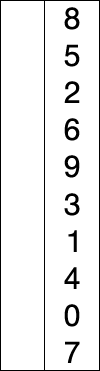# Selection Sort in C

Summary: this tutorial explains how the selection sort algorithm works and shows you how to implement the selection sort in C.

##Introduction to the selection sort algorithm

The selection sort is a simple sorting algorithm. The following illustrates the steps of the selection sort algorithm:

1. Find the minimum element in the list.
2. Swap it with the element in the first position of the list.
3. Repeat the steps above for all remaining elements of the list starting from the second position.

The complexity of the selection sort algorithm in the worst case is O(n2). The selection sort technique is less efficient on a large list. It generally performs worse than the insertion sort technique.

## Selection sort in C

The following is the selection sort in C implementation.

The following is the output of the selection sort program above:

In this tutorial, we have introduced you to selection sort algorithm and implemented the selection sort in C.